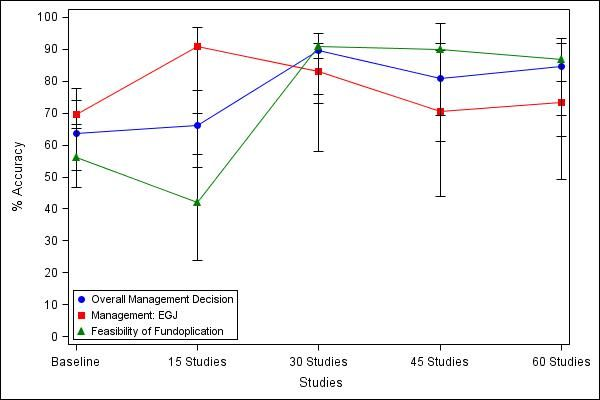## How to get accuracy projection (90%) based on existing values

Hi group,

I had generated SAS graph using existing data but I need help in getting projections/ estimations for number of studies required to touch approximately 90% accuracy. I would appreciate any help in this regard.

The data is about trainees reviewing number of studies to get maximum accuracy compared to gold standard value. The trainees reviewed 15 studies at a time, so I have data points baseline, 15 studies, 30 studies, etc. So I need to know how many studies are required to get Y-axis accuracy of 90%. I know that 90% has reached for one variable but I need to get projections for the other two and possibly indicate that trend in the graph I generated.

 Studies Mx Mx_low Mx_High MxEGJ MxEGJ_low MxEGJ_High Fundo Fundo_Low Fundo_High 0 63.6 46.7 77.9 69.6 65.2 73.9 56 52 66.5 15 66 53 77 91 70 97 42 24 57 30 89.5 76 92 83 58 87 91 73 95 45 81 61 92 70.5 44 89.5 90 69.3 98.2 60 84.6 62.6 91.9 73.3 49.2 80 86.7 69.3 93.32 REPLIES 2

## Re: How to get accuracy projection (90%) based on existing values

Does your "30 studies" result include the same studies in the "15 studies" and similar for the 45 or 60?

What were the rules for assigning studies to the groups?

What would you do if the data presented shows that the more studies included the "lower" the accuracy?

Which variable(s) are you using to determine the "accuracy"?

From the graph presented I think we also need to ask, have you brought this data into SAS yet?

One would also be tempted to ask how you define "90% accuracy" in terms of the basic data.

## Re: How to get accuracy projection (90%) based on existing values

Thanks for the reply. None of the studies included in 15 studies are part of 30 studies, and none from 30 studies are included in the 45, etc.

I am just reporting the accuracy trends for trainees compared to goldstandard results, so there is no specific answer to your question about what would I do if they lower accuracy...if they have lower accuaracy probaly they may need more studies to reach accurate diagnosis compared to goldstandard.

The graph was generated using SAS, so the data is imported to SAS from excel sheet for this purpose. On excel sheet for each variable I have accuracy values and its 95% CIs.

90% accuracy is when 90% of trainees agree with goldstandard diagnosis. 2x2 table.

Hope this helps. I am also attaching my excel sheet which I imported to SAS.

``````*Graphs;
%macro import (df, outname);
proc import datafile="&df"
out=&outname
dbms=EXCEL replace;
getnames=yes;
MIXED=NO;
SCANTEXT=YES;
USEDATE=YES;
SCANTIME=YES;
run;

%mend import;
*Ventrics Start and stop dates;
%import (E:\HRM\Graphs.xlsx, Graphs);

ods listing close;
ods graphics / reset width=600px height=400px imagename='Fig 1' imagefmt=jpeg;
ods html file='Surg.html' path='E:\HRM' style=journal;

/*ods html file='ap.html' path='C:\' style=journal; */
proc sgplot data=graphs;
scatter X=Studies Y=MP / yerrorlower=MP_LOW yerrorupper=MP_HIGH markerattrs=(color=blue symbol=CircleFilled size=8) name="scat" legendlabel="Motor Pattern";
series X=Studies Y=MP /  lineattrs=(color=blue pattern=1) ;
scatter X=Studies Y=BP / yerrorlower=BP_LOW yerrorupper=BP_HIGH markerattrs=(color=red symbol=SquareFilled size=8) name="scat1" legendlabel="Body Pattern";
series X=Studies Y=BP /  lineattrs=(color=red pattern=1) ;
scatter X=Studies Y=OutOb / yerrorlower=OutOb_LOW yerrorupper=OutOb_HIGH markerattrs=(color=green symbol=TriangleFilled size=8) name="scat2" legendlabel="Achalasia/ Outflow obstruction";
series X=Studies Y=OutOb /  lineattrs=(color=green pattern=1) ;

XAXIS TYPE = DISCRETE ;
YAXIS LABEL = '% Accuracy'    VALUES = (0 TO 100 BY 10);
keylegend "scat" "scat1" "scat2"  "scat4"  / position=bottomleft
across=1 location=inside ;
run;
ods html close;
ods listing;

ods listing close;
ods graphics / reset width=600px height=400px imagename='Fig 2' imagefmt=jpeg;
ods html file='Surg.html' path='E:\HRM' style=journal;

/*ods html file='ap.html' path='C:\' style=journal; */
proc sgplot data=graphs;
scatter X=Studies Y=Mx / yerrorlower=Mx_LOW yerrorupper=Mx_HIGH markerattrs=(color=blue symbol=CircleFilled size=8) name="scat" legendlabel="Overall Management Decision";
series X=Studies Y=Mx /  lineattrs=(color=blue pattern=1) ;
scatter X=Studies Y=MxEGJ / yerrorlower=MxEGJ_LOW yerrorupper=MxEGJ_HIGH markerattrs=(color=red symbol=SquareFilled size=8) name="scat1" legendlabel="Management: EGJ";
series X=Studies Y=MxEGJ /  lineattrs=(color=red pattern=1) ;
scatter X=Studies Y=Fundo / yerrorlower=Fundo_LOW yerrorupper=Fundo_HIGH markerattrs=(color=green symbol=TriangleFilled size=8) name="scat2" legendlabel="Feasibility of Fundoplication";
series X=Studies Y=Fundo /  lineattrs=(color=green pattern=1) ;

XAXIS TYPE = DISCRETE ;
YAXIS LABEL = '% Accuracy'    VALUES = (0 TO 100 BY 10);
keylegend "scat" "scat1" "scat2"  "scat4"  / position=bottomleft
across=1 location=inside ;
run;
ods html close;
ods listing;``````

Discussion stats
• 2 replies
• 716 views
• 0 likes
• 2 in conversation